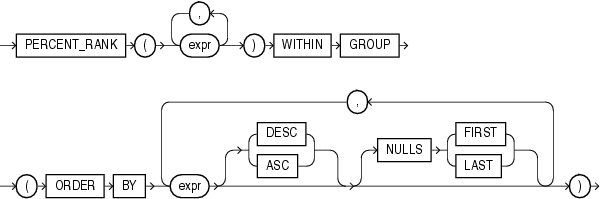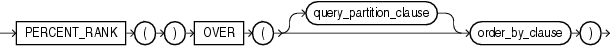## PERCENT_RANK

Aggregate Syntax

percent_rank_aggregate::=Description of the illustration percent_rank_aggregate.eps

Analytic Syntax

percent_rank_analytic::=Description of the illustration percent_rank_analytic.eps

for information on syntax, semantics, and restrictions

Purpose

`PERCENT_RANK` is similar to the `CUME_DIST` (cumulative distribution) function. The range of values returned by `PERCENT_RANK` is 0 to 1, inclusive. The first row in any set has a `PERCENT_RANK` of 0. The return value is `NUMBER`.

• As an aggregate function, `PERCENT_RANK` calculates, for a hypothetical row `r` identified by the arguments of the function and a corresponding sort specification, the rank of row `r` minus 1 divided by the number of rows in the aggregate group. This calculation is made as if the hypothetical row `r` were inserted into the group of rows over which Oracle Database is to aggregate.

The arguments of the function identify a single hypothetical row within each aggregate group. Therefore, they must all evaluate to constant expressions within each aggregate group. The constant argument expressions and the expressions in the `ORDER` `BY` clause of the aggregate match by position. Therefore the number of arguments must be the same and their types must be compatible.

• As an analytic function, for a row `r`, `PERCENT_RANK` calculates the rank of `r` minus 1, divided by 1 less than the number of rows being evaluated (the entire query result set or a partition).

Appendix C in Oracle Database Globalization Support Guide for the collation determination rules, which define the collation `PERCENT_RANK` uses to compare character values for the `ORDER` `BY` clause

Aggregate Example

The following example calculates the percent rank of a hypothetical employee in the sample table `hr.employees` with a salary of \$15,500 and a commission of 5%:

```SELECT PERCENT_RANK(15000, .05) WITHIN GROUP
(ORDER BY salary, commission_pct) "Percent-Rank"
FROM employees;

Percent-Rank
------------
.971962617```

Analytic Example

The following example calculates, for each employee, the percent rank of the employee's salary within the department:

```SELECT department_id, last_name, salary, PERCENT_RANK()
OVER (PARTITION BY department_id ORDER BY salary DESC) AS pr
FROM employees
ORDER BY pr, salary, last_name;

DEPARTMENT_ID LAST_NAME                     SALARY         PR
------------- ------------------------- ---------- ----------
10 Whalen                          4400          0
40 Mavris                          6500          0
Grant                           7000          0
. . .
80 Vishney                        10500 .181818182
80 Zlotkey                        10500 .181818182
30 Khoo                            3100         .2
. . .
50 Markle                          2200 .954545455
50 Philtanker                      2200 .954545455
50 Olson                           2100          1
. . .```